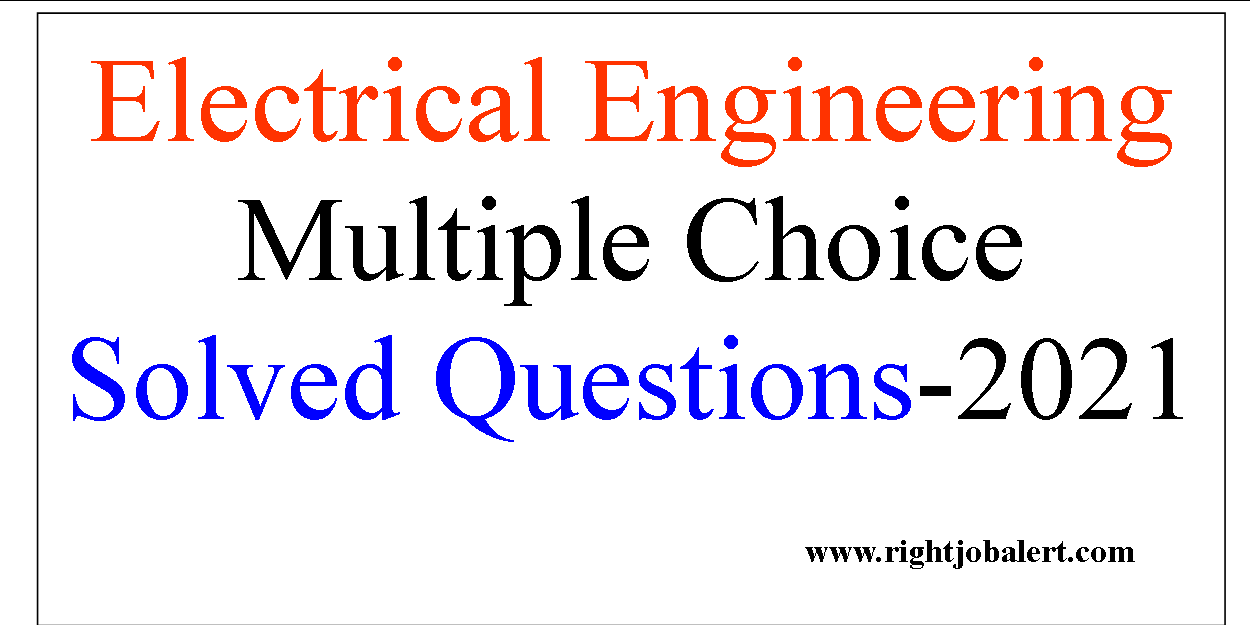# Electrical Engineering Multiple Choice Solved Questions-2021

The following Multiple Choice Electrical Engineering Questions are useful for those who are searching new government jobs in Public Sector and private sector companies. these questions are commonly asked during Electrical Engineers Recruitment written test or Online computer examinations. The Answers are provided below each questions for your easy learning.

Question  No. 1. The density of hydrogen is _____ times the density of air.
(a) 0.07
(b) 1.5
(c) 2.5
(d) 3.5Question  No. 2. A series generator can self-excite

(a) only if the load current is zero
(b) only if the load current is not zero
(c) irrespective of the value of load current
(d) none of the above

Question  No. 3. The terminal voltage of a series generator is 150 V when the load current is 5 A. If the
load current is increased to 10 A, the terminal voltage will be
(a) 150 V
(b) less than 150 V
(c) greater than 150 V
(d) none of the above

Question  No. 4. The mcrease in heat dissipation by air blasts is due to increase in
(a) conduction
(b) convection
(d) any ofthe above

#### Electrical Engineering Multiple Choice Solved Questions

Question  No. 5. The open circuit voltage of a compound generator is 250 V. At full load the terminal
voltage
(a) will be less than 250 V
(b) will always be 250 V
(c) may be greater or less than 250 V
(d) none of the above
Answer: Option: cQuestion  No. 6. The essential condition for parallel operation of two D.C. generators is that they have
‘(a) same kW rating
(b) the same operation r.p.m.
(c) the same drooping voltage charac-teristics
(d) same percentage regulation
Electrical Engineering Question
Question  No. 7. When two D.C. generators are running in parallel an equilizer bar is used
(a) to increase the series flux
(b) to increase the generated e.m.f.
(c) to reduce the combined effect of ar-mature reaction of both the machines
(d) so that the two identical machines will pass approximately equal currents to the load
Question  No. 8______is extensively used for those portions of magnetic circuit which carry steady flux
and need superior mechanical properties ?
(a) Grey cast-iron
(b) Cast steel
(c) High carbon steel
(d) Stainless steel
Ans: b
Question  No. 9. With a D.C. generator which of the following regulation is preferred ?
(a) 100% regulation
(b) infinite regulation
(c) 50% regulation
(d) 1% regulation
Question  No. 10. In a D.C. generator the critical resistance can be increased by
(a) increasing its field resistance
(b) decreasing its field resistance
(c) increasing its speed
(d) decreasing its speed